Related Articles

# Class 8 NCERT Solutions – Chapter 5 Data Handling – Exercise 5.2

• Last Updated : 15 Dec, 2020

### Problem 1. A survey was made to find the type of music that a certain group of young people liked in a city. The adjoining pie chart shows the findings of this survey. From this pie chart answer the following:

• If 20 people liked classical music, how many young people were surveyed?
• Which type of music is liked by the maximum number of people?
• If a cassette company were to make 1000 CD’s, how many of each type would they make?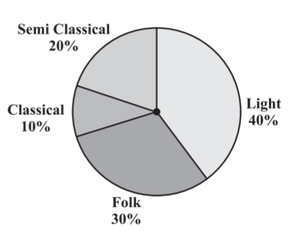Solution:

(i) Given, 20 people liked classical music. From the pie chart, we can see that classical music is liked by 10% of young people in the city. Let the total number of people in the city = x

Given, 10% of x = 20

(10/100) * x = 20

x = (20 * 100) / 10 = 200

So, 200 young people were surveyed.

(ii) Since light music occupies a maximum percentage (40%) in the pie chart, it is liked by the maximum number of people.

(iii) If a cassette company were to make 1000 CD’s, they would make

Classical music CDs = 10% of 1000

=  (10 / 100) * 1000

= 100 CDs

Semi Classical music CDs = 20% of 1000

= (20 / 100) * 1000

= 200 CDs

Folk music CDs = 30% of 1000

= (30 / 100) * 1000

= 300 CDs

Light music CDs = 40% of 1000

= (40 / 100) * 1000

= 400 CDs

### Problem 2. A group of 360 people was asked to vote for their favorite season from the three seasons rainy, winter, and summer.

• Which season got the most votes?
• Find the central angle of each sector.
• Draw a pie chart to show this information.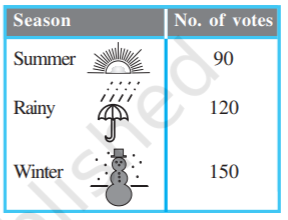Solution:

(i) Winter season got the most votes (150).

(ii) Find the proportion and percentage of each sector to calculate the central angle of each sector.

Total votes = 90 + 120 + 150 = 360

Proportion of summer season = 90 / 360 = 1 / 4, Percentage = (1 / 4) * 100 = 25%

Proportion of rainy season = 120 / 360 = 1 / 3, Percentage = (1 / 3) * 100 = 33.334%

Proportion of winter season = 150 / 360 = 5 / 12, Percentage = (5 / 12) * 100 = 41.667%

Now, the central angle of each sector is,

Summer season = (25 / 100) * 360° = 90°

Rainy season = (33.334 / 100) * 360° = 120°

Winter season = (41.667 / 100) * 360° = 150°

(iii) Pie Chart: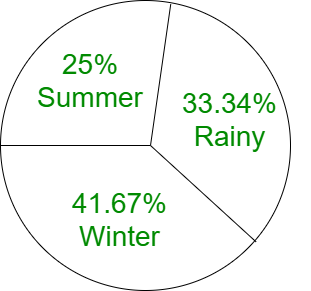### Problem 3. Draw a pie chart showing the following information. The table shows the colors preferred by a group of people.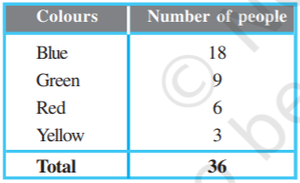Solution:

Find the proportion and percentage of each sector to calculate the central angle of each sector.

Total number of people = 18 + 9 + 6 + 3 = 36

Proportion of Blue = 18 / 36 = 1 / 2, Percentage = (1 / 2) * 100 = 50%

Proportion of Green = 9 / 36 = 1 / 4, Percentage = (1 / 4) * 100 = 25%

Proportion of Red = 6 / 36 = 1 / 6, Percentage = (1 / 6) * 100 = 16.667%

Proportion of Yellow = 3 / 36 = 1 / 12, Percentage = (1 / 12) * 100 = 8.334%

Now, the central angle of each sector is,

Blue = (50 / 100) * 360° = 180°

Green= (41.667 / 100) * 360° = 90°

Red = (16.667 / 100) * 360° = 60°

Yellow = (8.334 / 100) * 360° = 30°

Pie Chart: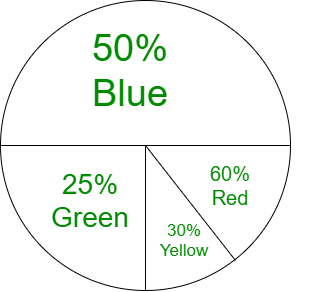### Problem 4. The adjoining pie chart gives the marks scored in an examination by a student in Hindi, English, Mathematics, Social Science, and Science. If the total marks obtained by the students were 540, answer the following questions.

• In which subject did the student score 105 marks?
• How many more marks were obtained by the student in Mathematics than in Hindi?
• Examine whether the sum of the marks obtained in Social Science and Mathematics is more than that in Science and Hindi.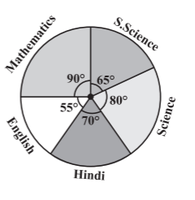Solution:

(i)  Given, total marks obtained by the students were 540, So,

For 540 marks, the central angle = 360°

For 1 mark, the central angle = (360 / 540)°

For 105 marks, the central angle = ((360 / 540) * 105)°

= 70°

From the given pie chart, 70° corresponds to Hindi language. Therefore, the student score 105 marks in   Hindi.

(ii) In mathematics, marks scored = (90 / 360) * 540

= 135 marks

In hindi, marks scored = (70 / 360) * 540

= 105 marks

Therefore, the student scored (135 – 105) = 30 marks more in Mathematics than in Hindi.

(iii) In mathematics, marks scored = 135 marks

In hindi, marks scored = 105 marks

In social science, marks scored  = (65 / 360) * 540

= 97.5 marks

In science, marks scored  = (80 / 360) * 540

= 120 marks

Marks in social science + mathematics = 97.5 + 135 = 232.5

Marks in science + hindi = 120 + 105 = 225

232.5 > 225

Yes, the sum of the marks obtained in Social Science and Mathematics is more than that in Science and     Hindi.

Alternative way:

Central angle of social science + mathematics =  65° + 90° = 155°

Central angle of science + hindi = 80° + 70° = 150°

155° > 150°

Yes, the sum of the marks obtained in Social Science and Mathematics is more than that in Science and Hindi.

### Problem 5. The number of students in a hostel, speaking different languages is given below. Display the data in a pie chart.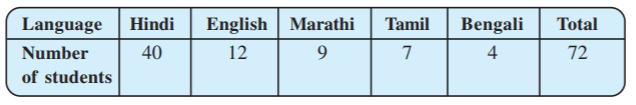Solution:

Find the proportion and percentage of each sector to calculate the central angle of each sector.

Total number of students = 72

Proportion of Hindi = 40 / 72 = 5 / 9, Percentage = (5 / 9) * 100 = 55.556%

Proportion of English = 12 / 72 = 1 / 6, Percentage = (1 / 6) * 100 = 16.667%

Proportion of Marathi = 9 / 72 = 1 / 8, Percentage = (1 / 8) * 100 = 12.5%

Proportion of Tamil = 7 / 72 = 7 / 72, Percentage = (7 / 72) * 100 = 9.723%

Proportion of Bengali = 4 / 72 = 1 / 18, Percentage = (1 / 18) * 100 = 5.556%

Now, the central angle of each sector is,

Hindi = (55.556 / 100) * 360° = 200°

English = (16.667 / 100) * 360° = 60°

Marathi = (12.5 / 100) * 360° = 45°

Tamil = (9.723 / 100) * 360° = 35°

Bengali = (5.556 / 100) * 360° = 20°

Pie Chart: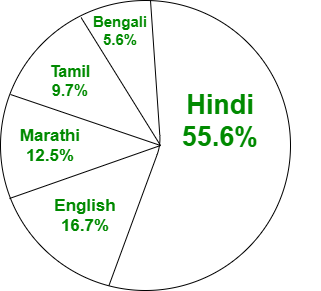Attention reader! All those who say programming isn’t for kids, just haven’t met the right mentors yet. Join the  Demo Class for First Step to Coding Coursespecifically designed for students of class 8 to 12.

The students will get to learn more about the world of programming in these free classes which will definitely help them in making a wise career choice in the future.

My Personal Notes arrow_drop_up